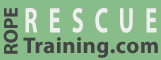# Calculating MA by Counting the Lines

The previous page explained how to calculate the mechanical advantage of a rope-and-pulley system using the T-System. This page explains the "counting the lines" method. "Counting the lines" is an easy to use method that works best with simple mechanical advantage systems, although this method can be used with compound systems.

## Simple Systems

To calculate the mechanical advantage by counting the lines, count the number of rope segments (aka "lines") that are either connected to the load or that are connected to a pulley that will travel at the same speed as the load.

Referring to this next illustration, three "lines" match this criteria, so this is a 3:1 system.

## Compound Systems

Calculating the mechanical advantage of compound rigging systems using the "counting the lines" method is a little challenging. As you may recall, a compound system is defined as one simple system pulling on another simple system. To calculate the mechanical advantage of these systems using the "counting the lines" method, you need to:

1. Isolate the simple systems within the compound system.
2. Use the "count the lines" method to determine the mechanical advantage of the individual simple systems.
3. Multiple the mechanical advantage of the individual systems to determine the overall mechanical advantage.